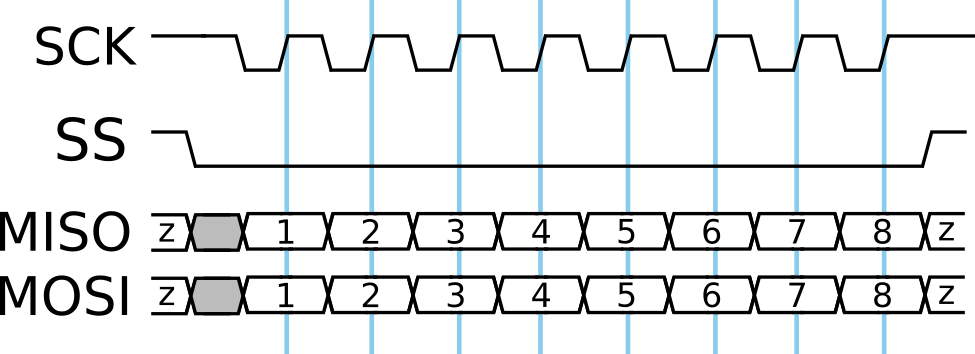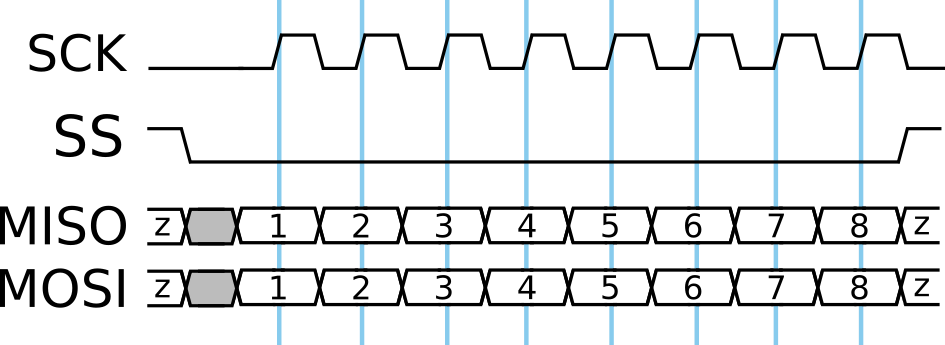After some months, here’s finally an article about a truly Embedded topic! We are going to take a quick look at the two basic parameters you want to carefully adjust when setting up an SPI bus: Clock Polarity (CPOL) and Clock Phase (CPHA).

SPI is a synchronous protocol. That means the data lines are sampled (and driven) at certain moments in time – in sync with a given clock line. For this to work, master and slave devices share a common understanding of how to interpret changes in the clock signal.

So, what information do we need to define clock characteristics? It’s a square wave (it’s supposed to be, at least), so it may be high, low, or transitioning between these two states.

On the receiving end, the data line is sampled on a certain edge of the clock signal. This is what makes the data synchronous to the clock, after all. Therefore, our first question could be: is this happening on the rising or falling edge?

This is what the Clock Phase (CPHA) attribute defines: 0 means first edge, 1 means second edge. Note that it doesn’t explicitly state rising or falling – it’s relative to the idle state of the clock line. Which brings us to the other attribute: Clock Polarity (CPOL). Without any ongoing transmission, the clock line can be either low (CPOL = 0) or high (CPOL = 1).

You see, we need both settings to fully define SPI communications. Let’s take a look at two examples (diagrams based on images from Wikipedia - SPI)!

The following plots show the clock (SCK), slave select (SS), and data lines over time. The moment where data is considered stable, and therefore valid, is marked with a vertical line. Take a close look at them to see if you can deduce CPOL and CPHA from the graphs (solutions below).

### Example #1:1. Data appears to be sampled on the rising clock edge.
2. To find out what this means for CPHA, we first need to determine CPOL.
3. CPOL appears to be 1 (idle high).
4. Therefore, CPHA is 1 (second edge).

### Example #2:1. Data appears to be sampled on the rising clock edge, just as before.
2. To find out what this means for CPHA, we first need to determine CPOL.
3. CPOL appears to be 0 (idle low).
4. Therefore, CPHA is 0 (first edge).

## Conclusion

We have seen that the two essential SPI clock settings are both needed, with one being relative to the other. If your SPI bus doesn’t work properly, you should definitely check the CPOL and CPHA settings!